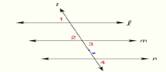Chapter 2.CT, Problem 16CTElementary Geometry For College St...

7th Edition
Alexander + 2 others
ISBN: 9781337614085

Solutions

Chapter
SectionElementary Geometry For College St...

7th Edition
Alexander + 2 others
ISBN: 9781337614085
Textbook Problem

In Exercises 16 and 18 , complete the missing statements or reasons for each proof. Given: ∠ 1 ≅ ∠ 2 ∠ 3 ≅ ∠ 4Prove: l ∥ n PROOF Statements Reasons 1. ∠ 1 ≅ ∠ 2 ∠ 3 ≅ ∠ 4 1.               _ 2.               _ 2. If two lines intersect, the vertical ∠ s are ≅ 3. ∠ 1 ≅ ∠ 4 3.               _ 4.               _ 5. If two lines are cut by a transversal so that a pair of alternate exterior ∠ s are ≅ , the lines are ∥

To determine

To prove:

The given statement.

Explanation

Given:

The following figure shows the given diagram.

1234

Properties:

(1) If two lines are cut by a transversal so that two corresponding angles are congruent, then these lines are parallel.

(2) If two lines intersect, then the vertically opposite angles formed are congruent.

Approach:

The first given statement is,

𝓁m

Line 𝓁 and line m are parallel lines and 1 and 2 are corresponding angles.

So,

12

2 and 3 are vertically opposite angles.

So,

23

Now, 12 and 23.

Use transitive property of congruence.

13

The given statement is,

34

Again, use transitive property of congruence.

14

1 and 4 are congruent corresponding angles.

Thus, 𝓁n.

 PROOF Statements Reasons 1.∠1≅∠2∠3≅∠4 1

Still sussing out bartleby?

Check out a sample textbook solution.

See a sample solution

The Solution to Your Study Problems

Bartleby provides explanations to thousands of textbook problems written by our experts, many with advanced degrees!

Get Started

Evaluate the integrals in Problems 1-26.

Mathematical Applications for the Management, Life, and Social Sciences

In Exercises 49-62, find the indicated limit, if it exists. 56. limx2x+2x2

Applied Calculus for the Managerial, Life, and Social Sciences: A Brief Approach

In Exercises 1-6, simplify the expression. (2)3+4(2)212

Calculus: An Applied Approach (MindTap Course List)

Fill in the blanks. Applying De Morgans Laws, we can write (ABC)c=.

Finite Mathematics for the Managerial, Life, and Social Sciences